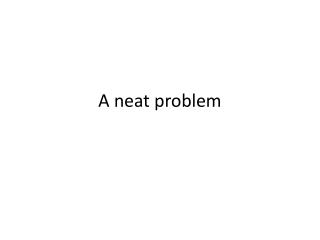DownloadDownload PresentationA neat problem

# A neat problem

Télécharger la présentation## A neat problem

- - - - - - - - - - - - - - - - - - - - - - - - - - - E N D - - - - - - - - - - - - - - - - - - - - - - - - - - -
##### Presentation Transcript

1. A neat problem

2. On the next slide there is a problem that has the initial statement: The town of Whoville has 100 identical consumers and 50 identical car washes. Each consumer has income of \$24. The diagram and chart below show the indifference curves of a typical consumer and the marginal cost curve of a typical car wash. Note the vertical axis should say All Other Goods.

3. To get the price of a car wash today: Note in the graph if all \$24 of the consumer is spent on car washes then we see 12 car washes could be purchased. On this budget line it means the price of a wash is \$2. We see the typical individual would actually take 4 washes at a price of \$2. The quantity demanded would be 4 times 100 = 400 washes. If the price is \$2 the firm would have MC = MR at a Q less than 1. With 50 firms the quantity supplied would be less than 50. With Qd > Qs price will rise. If the price rises to \$3, note if all \$24 of the consumer is spent on car washes then 8 car washes could be purchased. We see the typical individual would actually take 3 washes at a price of \$3. The quantity demanded would be 3 times 100 = 300 washes. If the price is \$3 the firm would have MC = MR at a Q = 1. With 50 firms the quantity supplied would be 50. With Qd > Qs price will rise. If the price rises to \$6, note if all \$24 of the consumer is spent on car washes then 4 car washes could be purchased. We see the typical individual would actually take 2 washes at a price of \$6. The quantity demanded would be 2 times 100 = 200 washes. If the price is \$6 the firm would have MC = MR at a Q = 4. With 50 firms the quantity supplied would be 200. With Qd = Qs we see the price in the market = 6.

4. Part b If there is no entry or exit then it must be that economic profit = 0. With revenue of \$24 (which is \$6 times 4 units) and variable costs of 3 + 4 + 5 + 6 = \$18, The fixed cost must be \$24 - \$18 = \$6.